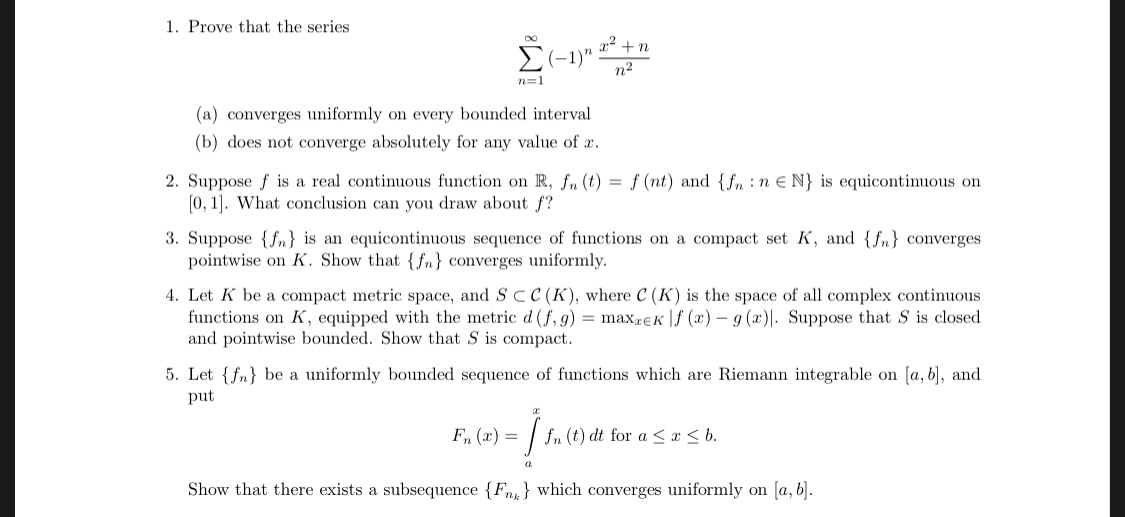1. Prove that the series7n(a) converges uniformly on every bounded interval(b) does not converge absolutely for any value of r2. Suppose f is a real continuous function on R, n (t) (nt) and { n :n N) is equicontinuous on3. Suppose is an equicontinuous sequence of functions on a compact set K, and {fn) converges. Let K be a compact metric space, and S CC(K), where C (K) is the space of all complex continuous0,1. What conclusion can you draw about f?pointwise on K. Show that {fn converges uniformlyfunctions on K, equipped with the metric d ( .9) = max EK | (x)-g(z) . Suppose that s is closedand pointwise bounded. Show that S is compact5. Let {fn be a uniformly bounded sequence of functions which are Riemann integrable on [a, b, andput岔Fn (x)/fn (t) dt for a ssb0Show that there exists a subsequence {Fn^1 which converges uniformly on [a, b

Questionhelp_outlineImage Transcriptionclose1. Prove that the series 7n (a) converges uniformly on every bounded interval (b) does not converge absolutely for any value of r 2. Suppose f is a real continuous function on R, n (t) (nt) and { n :n N) is equicontinuous on 3. Suppose is an equicontinuous sequence of functions on a compact set K, and {fn) converges . Let K be a compact metric space, and S CC(K), where C (K) is the space of all complex continuous 0,1. What conclusion can you draw about f? pointwise on K. Show that {fn converges uniformly functions on K, equipped with the metric d ( .9) = max EK | (x)-g(z) . Suppose that s is closed and pointwise bounded. Show that S is compact 5. Let {fn be a uniformly bounded sequence of functions which are Riemann integrable on [a, b, and put 岔 Fn (x)/fn (t) dt for a s sb 0 Show that there exists a subsequence {Fn^1 which converges uniformly on [a, b fullscreen
Step 1

As per norms . three questions ,1a, 1b and 2 are answered. To analyze various aspects of convergence of the given series.

Step 2

1a)Proof that the given series converges for any real x (using Leibniz test on alternating series)

Step 3

1a) Proof that the series is actually uniformly convergent provided x is in a bounded interval ...

Want to see the full answer?

See Solution

Want to see this answer and more?

Our solutions are written by experts, many with advanced degrees, and available 24/7

See Solution
Tagged in

Math